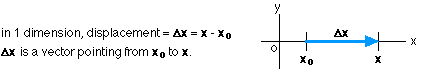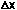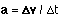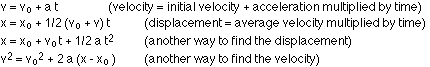## Motion in one dimension

9-8-99

Sections 2.1 - 2.5

### Motion in 1 dimension

We live in a 3-dimensional world, so why bother analyzing 1-dimensional situations? Basically, because any translational (straight-line, as opposed to rotational) motion problem can be separated into one or more 1-dimensional problems. Problems are often analyzed this way in physics; a complex problem can often be reduced to a series of simpler problems.

The first step in solving a problem is to set up a coordinate system. This defines an origin (a starting point) as well as positive and negative directions. We'll also need to distinguish between scalars and vectors. A scalar is something that has only a magnitude, like area or temperature, while a vector has both a magnitude and a direction, like displacement or velocity.

In analyzing the motion of objects, there are four basic parameters to keep track of. These are time, displacement, velocity, and acceleration. Time is a scalar, while the other three are vectors. In 1 dimension, however, it's difficult to see the difference between a scalar and a vector! The difference will be more obvious in 2 dimensions.

### Displacement

The displacement represents the distance traveled, but it is a vector, so it also gives the direction. If you start in a particular spot and then move north 5 meters from where you started, your displacement is 5 m north. If you then turn around and go back, with a displacement of 5 m south, you would have traveled a total distance of 10 m, but your net displacement is zero, because you're back where you started. Displacement is the difference between your final position (x) and your starting point (xo) :### Speed and velocity

Imagine that on your way to class one morning, you leave home on time, and you walk at 3 m/s east towards campus. After exactly one minute you realize that you've left your physics assignment at home, so you turn around and run, at 6 m/s, back to get it. You're running twice as fast as you walked, so it takes half as long (30 seconds) to get home again.

There are several ways to analyze those 90 seconds between the time you left home and the time you arrived back again. One number to calculate is your average speed, which is defined as the total distance covered divided by the time. If you walked for 60 seconds at 3 m/s, you covered 180 m. You covered the same distance on the way back, so you went 360 m in 90 seconds.

Average speed = distance / elapsed time = 360 / 90 = 4 m/s.

The average velocity, on the other hand, is given by:

Average velocity = displacement / elapsed time.

In this case, your average velocity for the round trip is zero, because you're back where you started so the displacement is zero

We usually think about speed and velocity in terms of their instantaneous values, which tell us how fast, and, for velocity, in what direction an object is traveling at a particular instant. The instantaneous velocity is defined as the rate of change of position with time, for a very small time interval. In a particular time interval delta t, if the displacement is, the velocity during that time interval is:The instantaneous speed is simply the magnitude of the instantaneous velocity.

### Acceleration

An object accelerates whenever its velocity changes. Going back to the example we used above, let's say instead of instantly breaking into a run the moment you turned around, you steadily increased your velocity from 3m/s west to 6 m/s west in a 10 second period. If your velocity increased at a constant rate, you experienced a constant acceleration of 0.3 m/s per second (or, 0.3 m/s2).

We can figure out the average velocity during this time. If the acceleration is constant, which it is in this case, then the average velocity is simply the average of the initial and final velocities. The average of 3 m/s west and 6 m/s west is 4.5 m/s west. This average velocity can then be used to calculate the distance you traveled during your acceleration period, which was 10 seconds long. The distance is simply the average velocity multiplied by the time interval, so 45 m.

Similar to the way the average velocity is related to the displacement, the average acceleration is related to the change in velocity: the average acceleration is the change in velocity over the time interval (in this case a change in velocity of 3 m/s in a time interval of 10 seconds). The instantaneous acceleration is given by:As with the instantaneous velocity, the time interval is very small (unless the acceleration is constant, and then the time interval can be as big as we feel like making it).

On the way out, you traveled at a constant velocity, so your acceleration was zero. On the trip back your instantaneous acceleration was 0.3 m/s2 for the first 10 seconds, and then zero after that as you maintained your top speed. Just as you arrived back at your front door, your instantaneous acceleration would be negative, because your velocity drops from 6 m/s west to zero in a small time interval. If you took 2 seconds to come to a stop, your acceleration is -6 / 2 = -3 m/s2.

### Kinematics equations when the acceleration is constant

When the acceleration of an object is constant, calculations of the distance traveled by an object, the velocity it's traveling at a particular time, and/or the time it takes to reach a particular velocity or go a particular distance, are simplified. There are four equations that can be used to relate the different variables, so that knowing some of the variables allows the others to be determined.

Note that the equations apply under these conditions:

1. the acceleration is constant
2. the motion is measured from t = 0
3. the equations are vector equations, but the variables are not normally written in bold letters. The fact that they are vectors comes in, however, with positive and negative signs.

The equations are:The equations above are all derived in section 2.5.